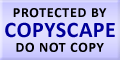Submit Assignment From Here
Name
:
Email
:
Phone
:
Country
:
Willing to pay US\$
:
Subject
:
Topic
:
Levels
:
:Hours
:

# Bivariate Regression Homework Help

Bivariate regression is a simple linear regression. Bivariate Regression is the simple because the model explores the relationship between two variables and it examines only linear relationships of the two selected variables. It tries to develop an interdependent relationship among the two variables. Its main focus is to predict one variable with the help of information available from the second variable. The purpose of Bivariate Regression is to test hypotheses, make predictions, allow for multivariate analysis, find a rate which changes the variables etc. So, Bivariate Regression function interprets change in one variable as change in value of second variable. Bivariate Regression names the variables as Criterion variable and predictor variable. Predictor variable is the used to forecast the changes in criterion variable. On the other hand criterion variable is the variable whose variations which we want to forecast. In Bivariate Regression the most important criterion is to find out the BLUE. The BLUE is the best linear UN – bias estimator. We can put it up as the criterion which has the minimum errors, which can form a relationship with other variables, in the criterion the parameter truly reflects the effects on it, and the estimator should be present.US